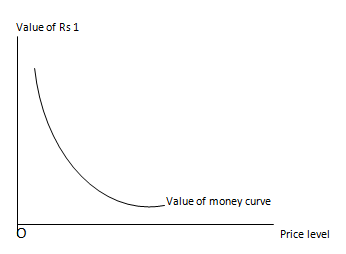# Value of money

The value of money is defines as the quantity of goods and services that can be purchased with the amount of money. It is given by the ratio of amount of money and general price level.

Mathematically,
value of money = amount of money / price level

The value of re 1 is the inverse of general price level.

Mathematically,

value of re. 1 = 1 / p

Value of money is inversely related to price level. If price level rises, the value of money decreases and vice versa

If P ↑, value of money ↓
If P ↓, value of money ↑

The relationship between value of money and price level can be explained with the help of table and figure a following

 Price level (P) Value of re. 1 ( 1/P) Rs 10 0.1 Rs 20 0.05 Rs 30 0.033

In the above table when price level is increased from Rs 10 to Rs 20, 30 value of re 1 is decreased from 0.1 units to 0.05 and 0.033 unit respectively. It shows the inverse relationship between value of money and price level. It we represent the value of money with respect to price level we obtain a monotonically downward sloped curve as shown below:In the above figure, the convex curve shows the relationship between value of money and price level. It is downward sloped which shoes that value of Rs1 decreases with every increase in price level.